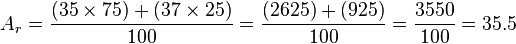# Atomic mass facts for kids

Kids Encyclopedia Facts

An atomic mass (symbol: ma) is the mass of a single atom of a chemical element. It includes the masses of the 3 subatomic particles that make up an atom: protons, neutrons and electrons.

Atomic mass can be expressed in grams. However, because each atom has a very small mass, this is not very helpful. Instead, atomic mass is expressed in unified atomic mass units (unit symbol: u). 1 atomic mass unit is defined as 1/12 of the mass of a single carbon-12 atom. 1 u has a value of 1.660 539 066 60(50) × 10−27 kg.

A carbon-12 atom has a mass of 12 u. Because electrons are so light, we can say that the mass of a carbon-12 atom is made of 6 protons and 6 neutrons. Because the masses of protons and neutrons are almost exactly the same, we can say that both protons and neutrons have a mass of roughly 1 u. Hence, we can get a rough value of an atom's mass in atomic mass units by working out the sum of the number of protons and the number of neutrons in the nucleus, which is called the mass number. The atomic mass of an atom is usually within 0.1 u of the mass number.

The number of protons an atom has determines what element it is. However, most elements in nature consist of atoms with different numbers of neutrons. An atom of an element with a certain number of neutrons is called an isotope. For example, the element chlorine has two common isotopes: chlorine-35 and chlorine-37. Both isotopes of chlorine have 17 protons, but chlorine-37 has 20 neutrons, 2 more neutrons than chlorine-35, which has 18. Each isotope has its own atomic mass, called its isotopic mass. In the case of chlorine, chlorine-35 has a mass of around 35 u, and chlorine-37 around 37 u.

As mentioned above, note that the atomic mass of an atom is not the same as its mass number. The mass number (symbol: A) of an atom is the sum of the number of protons and the number of neutrons in the nucleus. Mass numbers are always whole numbers with no units. Also, relative isotopic mass is not the same as isotopic mass, and relative atomic mass (also called atomic weight) is not the same as atomic mass.

A relative isotopic mass is the mass of an isotope relative to 1/12 of the mass of a carbon-12 atom. In other words, a relative isotopic mass tells you the number of times an isotope of an element is heavier than one-twelfth of an atom of carbon-12. The word relative in relative isotopic mass refers to this scaling relative to carbon-12. Relative isotopic mass is similar to isotopic mass and has exactly the same numerical value as isotopic mass, whenever isotopic mass is expressed in atomic mass units. However, unlike isotopic mass, relative isotopic mass values have no units.

Like relative isotopic mass, a relative atomic mass (symbol: Ar) is a ratio with no units. A relative atomic mass is the ratio of the average mass per atom of an element from a given sample to 1/12 the mass of a carbon-12 atom. We find the relative atomic mass of a sample of an element by working out the abundance-weighted mean of the relative isotopic masses. For example, to continue the chlorine example from above, if there is 75% of chlorine-35 and 25% of chlorine-37 in a sample of chlorine,$A_r = \frac{(35\times75)+(37\times25)}{100} = \frac{(2625)+(925)}{100} = \frac{3550}{100} = 35.5$

## Related pagesAtomic mass Facts for Kids. Kiddle Encyclopedia.